3.3. Dynamics of barred galaxies

The central problem in the dynamics of spiral galaxies is the understanding of the origin and evolution of the spiral and barred structures that appear with a range of shapes in these partly stellar, partly gaseous systems, where young stars are continuously being born out of the gas. In particular, these structures appear to be long-lived in spite of the presence of a shearing velocity field where the angular velocity of rotation varies with distance from the centre. This led to the idea that the observed structure is the manifestation of a density wave rather than a material configuration.

In the early 1960s B. Lindblad (1963, 1964) suggested a circulation theory of spiral structure, where due to the gravitational forces of the spiral arms matter would circulate between the arms and create a self-supporting quasi-permanent spiral structure. Lin and Shu (1964) linearized the problem and formulated a theory for self-consistent tightly wound spiral stellar density waves. (For a recent review of the density wave theory see Bertin & Lin 1996.) This triggered a large amount of work in this field (see Toomre 1977 for a review) and a picture emerged where the circulating interstellar matter was strongly compressed in the potential well of the spiral arms and there created young stellar associations which ionize the gas to form H II regions and then leave the arms with increasing age. The extent of the spiral structure was governed by a series of resonances between orbital motion and the rotating potential.

However, the barred galaxies pose a somewhat different problem and, as a matter of fact, it seems that when considering the origin and maintenance of structures of galaxies our understanding is more complete for the barred galaxies. Sellwood and Wilkinson (1993) have reviewed in a very comprehensive way our present understanding of the dynamics of barred galaxies. Numerous numerical N-body simulations have shown that a rotationally supported stellar disk of a certain flatness is strongly unstable to the formation of a bi-symmetric stellar bar running through the centre and rotating with a certain pattern angular velocity. These numerical results are supported by stability analyses which lead to the study of normal modes of an axisymmetric stellar disk. The dominant modes found in these studies grow fast and have an open bi-symmetric S-shape. The numerical N-body simulations show that this modal bar straightens up and as time goes on ends just inside the Lagrange points on the bar major axis (defining the so called co-rotation radius), while the originally formed weak trailing spiral arms extending from the ends of the bar are transient and fade away. It seems likely that the formation of the disk and the bar are not two separate events, as assumed in most simulations, but are in fact intimately coupled (Sellwood & Wilkinson 1993). As suggested by Ostriker and Peebles (1973) a massive halo may check this bar instability. However, as pointed out by Sellwood and Wilkinson, there are reasons why this checking mechanism does not seem to work in spiral galaxies.

The bar potential affects the dynamics of the rest of the system and there are numerous studies of orbits in rotating bar potentials. The majority of stars within the bar seem to follow epicyclic orbits, trapped around elongated closed (in the frame rotating with the bar) periodic orbits aligned with the bar. The gaseous component, due to internal friction, reacts in a different way. When gas pressure is neglected, the gas stream lines must nearly coincide with the stellar periodic orbits. Gas stream lines are not permitted to intersect and large scale shocks may develop when the perturbing potential becomes strong. As shown by hydrodynamical calculations (e.g. Sanders & Huntley 1976) the flow pattern settles down to a density wave which is steady in the rotating frame of the bar. The dominating shape is a trailing spiral structure, which is governed by the series of main resonances in the system.

To understand the nature of these resonances, let us assume that the differential rotation of the disk in the presence of a weak bar is described by the circular angular velocity as a function of the radius(R) and that the perturbing bar potential rotates as a solid body with the pattern angular velocityp. The most fundamental resonance occurs where the circular angular velocity equals the angular velocity of the bar, i.e.=p. This is the corotation resonance.

In a non-circular orbit a particle oscillates in the radial direction with a frequency, which we will denote by, and which is a function of the mean radius and the amplitude of the radial motion. Such an orbit can always be transferred into a closed periodic orbit by introducing a rotating coordinate system. If we choose the closed orbit to have m apocentra, the angular velocity of this coordinate system is-/m or+/m, depending on whether the particle should describe the closed orbit in the direct or retrograde direction. For a bar with its two-fold symmetry the fundamental resonances occur when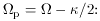the so-called Inner Lindblad (1) Resonance (ILR),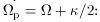the so-called Outer Lindblad Resonance (OLR).

Resonances with values of m other than 2 will arise due to other order Fourier components in the development of the bar potential. Such resonances also arise due to the non-linear nature of the problem (so called ultra- or hyper-harmonic resonances).

If the radial amplitude is small,can be considered as a function of R only. Fig. 13 shows the rotation curve, i.e. circular velocity as a function of R, which we later will adopt for NGC 1365, as well as the functionsand°/m, where m = 2, 4, and 6. The horizontal line, representing the pattern angular velocityp, then gives the positions of the corresponding resonances.

The prime effect of the bar on stellar orbits is to enhance their radial amplitudes, in particular in the neighbourhood of resonances. In the case of m = 2, closed stellar orbits will be elongated along the bar or at right angles to the bar depending on the position with respect to the resonances and the relative rate of radial change of the bar potential. At each resonance the phase of the oscillation and the orientation of the orbit in this case will shift by/2 with respect to the bar (in analogy with the forced oscillation of a pendulum, where the phase of the oscillation shifts byat the resonance frequency). If, however, dissipative forces are present as in the gas dynamical case, the amplitudes will be damped and the orientation of the closed orbits of the gas will deviate from that of the bar and gradually twist when passing over a resonance and thereby form a spiral density wave pattern.

We will give a more mathematical illustration as follows using the epicyclic approximation with some simplifying assumptions (see Lindblad & Lindblad 1994; Wada 1994). Consider an orbit that does not deviate much from a circular orbit with radius Ro, and introduce a coordinate system rotating with the angular velocity of circular motion(Ro). The deviation from circular motion at R = Ro is given by the coordinatesin the radial direction andalong the circular arc with radius Ro. We assume a perturbing bisymmetric bar potential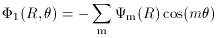where m only takes even values.is the angular distance from the bar potential minimum. This bar is supposed to rotate with the angular velocityp, and we introducem(R) as the frequency with which a particle in circular orbit encounters the potential minimum of a certain Fourier component, i.e.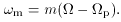(1)

To simulate gas dynamics we introduce a frictional force proportional to the deviation from circular motion at the position of the particle and with a damping coefficient. Assuming that we are not close to corotation the equations of motion in the linear epicyclic approximation are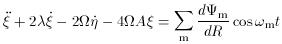(2)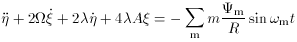(3)

where Oort's constant A = -1/2 R d/ dR. All quantities dependent on R are to be evaluated at R = Ro. We choose Ro to be the radius of the circular orbit described by the guiding centre of the final orbit. Further we assume thatis small and that we are close to a resonance, i.e. (2 -m2) is small. We then get the solution to Eqs. (2) and (3) after oscillations around the central orbit have damped out due to friction: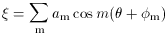(4)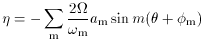(5)

where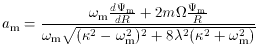and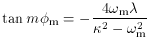The solution given by (4) and (5) represents the forced oscillation due to the perturbing potential. Asis small, the amplitudes get large form, and the terms with the corresponding value of m will dominate the solutions. The equations describe an orbit closed in a frame rotating with the perturbing potential and an orientation with respect to the bar determined by the phase anglem. Realising from Eq. (1) that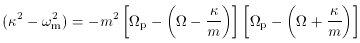we see that the amplitudes get large for the resonance conditions for the ILR and OLR mentioned above. Also, when passing across a resonance the orientation of the closed orbit turns smoothly by close to 180°/m.

This is illustrated in Fig. 12 where the exact solution to Eqs. (2) and (3) has been used and the bi-symmetric bar has been modeled from the bar of NGC 1365 (see Section 3.4). The axisymmetric component of the potential, however, is chosen to give a strictly flat rotation curve with a clearcut single ILR at a distance of 6.7 kpc from the centre. As can be seen, the innermost elongated gas orbits are oriented close to perpendicular to the (horizontal) bar. When approaching the ILR the amplitude increases and the orbits twist in the counter-clockwise direction until outside the resonance they tend to be elongated along the bar. The result is a trailing spiral density wave pattern. With increasing strength of the bar, the amplitudes increase until the orbits touch and large-scale shocks develop along the leading side of the bar.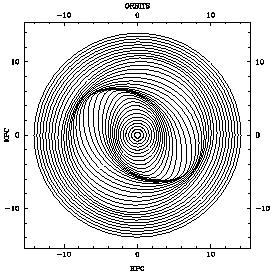Figure 12. Closed gas orbits in a barred potential computed analytically in the epicyclic approximation. The bar is horizontal and the rotation is clockwise. The ILR is at 6.7 kpc from the centre.

Lindblad and Lindblad (1994) as well as Wada (1994) compared the results given by the linear epicyclic approximation with numerical hydrodynamic calculations demonstrating the general agreement. The linear approximation is a useful tool to give insight into the orbital structures and to very quickly scan the multi-dimensional parameter space. However, this simple approximation fails around corotation where closed stellar orbits tend to circulate around Lagrange points and the gas response seems to be rather dependent on the self-gravity of the gas (Section 3.4).

Sellwood and Sparke (1988) introduce a complication to this picture of a completely bar driven spiral structure. They note that in a number of N-body simulations an outer spiral structure appears with a pattern speed much lower than that of the bar. They argue that barred galaxies with well-developed outer spiral structure must have spiral density waves in the stellar component which are driven by some other mechanism than a steady bar and thus could well have a pattern speed different from that of the bar. As far as observations go they mention NGC 1365 as giving supporting evidence and Sellwood and Wilkinson (1993) even state that the most convincing evidence for two separate pattern speeds in a galaxy is given by NGC 1365. P.A.B. Lindblad et al. (1996) find no need for two pattern speeds in NGC 1365 and we will further discuss this question in Section 3.4.

1 Named after Bertil Lindblad 1895-1965 Back.